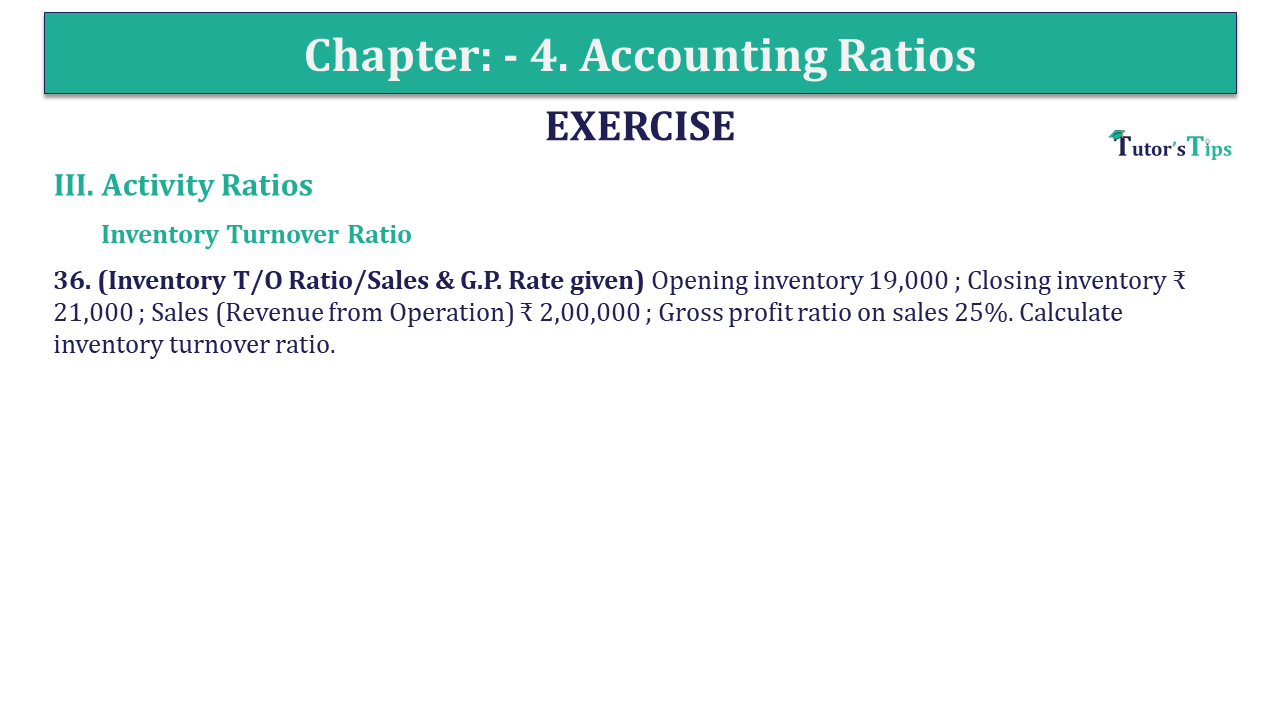# Question 36 Chapter 4 of +2-B – USHA Publication 12 ClassQ-36- CH-4 Book 2 - Usha Pub. +2 Book 2020 - Solution

Question 36 Chapter 4 of +2-B

36. (Inventory T/O Ratio/Sales & G.P. Rate given) Opening inventory 19,000 ; Closing inventory ₹ 21,000 ; Sales (Revenue from Operation) ₹ 2,00,000 ; Gross profit ratio on sales 25%. Calculate inventory turnover ratio.

## The solution of Question 36 Chapter 4 of +2-B: –

 (i) Debt Equity Ratio = Cost of goods sold Average Inventory

 Total Long term Debt = Sales – Gross profit = ₹ 2,00,000 – ₹ 50,000* = ₹ 1,50,000
 Debt Equity Ratio = Opening Inventory + Closing Inventory 2 = ₹ 19,000 + ₹ 21,000 2 = ₹ 20,000

 Inventory Turnover Ratio = ₹ 1,50,000 ₹ 20,000 = 7.5 Times

 *Gross profit = 25% of Sales Sales = ₹ 2,00,000
 Gross profit = ₹ 2,00,000 X 25 100 = ₹ 50,000

Also, Check out the solved question of previous Chapters: –